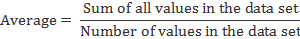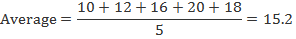Chapter 1

# AVERAGES

Introduction

Anaverage is the most likely middle value of a data set. It is the mean value ofa data set around which all the numbers are clustered and hence isrepresentative of the set. An average is the value that each element of the setwould take if all the elements of the set were to be the same. The average ofthe elements of a set can be calculated as the sum of all values in the setdivided by the total number of values.Anaverage is a useful indicator when the number of elements in a particular setis very large. In such a case, the average reduces the set to a single valuewhich becomes the representative of the set. For instance, if a player hasplayed 400 matches and has scored 16950 runs in those matches, then that player'sperformance can be expressed as a single statistic i.e. batting average. Thisis calculated by dividing 16950 by 400 to give 42.375 or 42.38. This impliesthat the player can be expected to score approximately 42 runs every time hebats.

Thus,an average is a comparative tool to compare multiple sets when the objects inthe different sets are similar in nature.

However,an average can be misleading if the number of samples is small and/or the valueof the samples is very spread out. For instance, if another player has playedonly 3 matches and has scored 0, 1 and 149 in the three matches respectively,his average becomes (0 + 1 + 149)/3 = 50 runs. As per this average, the playeris now expected to score 50 runs in every match which is clearly not the case.

Example:

Agroup of 5 friends scored 10, 12, 16, 20 and 18 in a class test. Find theaverage score of the students.

Solution:View All Jobs